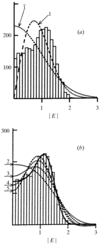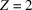International
Tables for
Crystallography
Volume B
Reciprocal space
Edited by U. Shmueli

International Tables for Crystallography (2006). Vol. B. ch. 2.1, p. 199   | 1 | 2 |

## Section 2.1.7.1. Introduction

U. Shmuelia* and A. J. C. Wilsonb

aSchool of Chemistry, Tel Aviv University, Tel Aviv 69 978, Israel, and bSt John's College, Cambridge, England
Correspondence e-mail:  ushmueli@post.tau.ac.il

#### 2.1.7.1. Introduction

| top | pdf |

The probability density functions (p.d.f.'s) of the magnitude of the structure factor, presented in Section 2.1.5, are based on the central-limit theorem discussed above. In particular, the centric and acentric p.d.f.'s given by equations (2.1.5.11)and (2.1.5.8), respectively, are expected to account for the statistical properties of diffraction patterns obtained from crystals consisting of nearly equal atoms, which obey the fundamental assumptions of uniformity and independence of the atomic contributions and are not affected by noncrystallographic symmetry and dispersion. It is also assumed there that the number of atoms in the asymmetric unit is large. Distributions of structure-factor magnitudes which are based on the central-limit theorem, and thus obey the above assumptions, have been termed ideal', and the subjects of the following sections are those distributions for which some of the above assumptions/restrictions are not fulfilled; the latter distributions will be called non-ideal'.

We recall that the assumption of uniformity consists of the requirement that the fractional part of the scalar productbe uniformly distributed over the [0, 1] interval, which holds well ifare rationally independent (Hauptman & Karle, 1953), and permits one to regard the atomic contribution to the structure factor as a random variable. This is of course a necessary requirement for any statistical treatment. If, however, the atomic composition of the asymmetric unit is widely heterogeneous, the structure factor is then a sum of unequally distributed random variables and the Lindeberg–Lévy version of the central-limit theorem (cf. Section 2.1.4.4) cannot be expected to apply. Other versions of this theorem might still predict a normal p.d.f. of the sum, but at the expense of a correspondingly large number of terms/atoms. It is well known that atomic heterogeneity gives rise to severe deviations from ideal behaviour (e.g. Howells et al., 1950) and one of the aims of crystallographic statistics has been the introduction of a correct dependence on the atomic composition into the non-ideal p.d.f.'s [for a review of the early work on non-ideal distributions see Srinivasan & Parthasarathy (1976)]. A somewhat less well known fact is that the dependence of the p.d.f.'s ofon space-group symmetry becomes more conspicuous as the composition becomes more heterogeneous (e.g. Shmueli, 1979; Shmueli & Wilson, 1981). Hence both the composition and the symmetry dependence of the intensity statistics are of interest. Other problems, which likewise give rise to non-ideal p.d.f.'s, are the presence of heavy atoms in (variable) special positions, heterogeneous structures with complete or partial noncrystallographic symmetry, and the presence of outstandingly heavy dispersive scatterers.

The need for theoretical representations of non-ideal p.d.f.'s is exemplified in Fig. 2.1.7.1(a), which shows the ideal centric and acentric p.d.f.'s together with a frequency histogram ofvalues, recalculated for a centrosymmetric structure containing a platinum atom in the asymmetric unit of(Faggiani et al., 1980). Clearly, the deviation from the Gaussian p.d.f., predicted by the central-limit theorem, is here very large and a comparison with the possible ideal distributions can (in this case) lead to wrong conclusions.Figure 2.1.7.1 | top | pdf |Atomic heterogeneity and intensity statistics. The histogram appearing in (a) and (b) was constructed fromvalues which were recalculated from atomic parameters published for the centrosymmetric structure of C6H18Cl2N4O4Pt (Faggiani et al., 1980). The space group of the crystal is,, i.e. all the atoms are located in general positions. (a) A comparison of the recalculated distribution ofwith the ideal centric [equation (2.1.5.11)] and acentric [equation (2.1.5.8)] p.d.f.'s, denoted byand 1, respectively. (b) The same recalculated histogram along with the centric correction-factor p.d.f. [equation (2.1.7.5)], truncated after two, three, four and five terms (dashed lines), and with that accurately computed for the correct space-group Fourier p.d.f. [equations (2.1.8.5)and (2.1.8.22)] (solid line).

Two general approaches have so far been employed in derivations of non-ideal p.d.f.'s which account for the above-mentioned problems: the correction-factor approach, to be dealt with in the following sections, and the more recently introduced Fourier method, to which Section 2.1.8is dedicated. In what follows, we introduce briefly the mathematical background of the correction-factor approach, apply this formalism to centric and acentric non-ideal p.d.f.'s, and present the numerical values of the moments of the trigonometric structure factor which permit an approximate evaluation of such p.d.f.'s for all the three-dimensional space groups.

### ReferencesFaggiani, R., Lippert, B. & Lock, C. J. L. (1980). Heavy transition metal complexes of biologically important molecules. 4. Crystal and molecular structure of pentahydroxonium chloro(uracilato-N(1))(ethylenediamine)platinum(II)chloride (H5O2)[PtCl(NH2CH2CH2NH2)(C4H5N2O2)]Cl, and chloro(thyminato-N(1))(ethylenediamine)platinum(II), PtCl(NH2CH2CH2NH2)(C5H5N2O2). Inorg. Chem. 19, 295–300.Google ScholarHauptman, H. & Karle, J. (1953). Solution of the phase problem. I. The centrosymmetric crystal. Am. Crystallogr. Assoc. Monograph No. 3. Dayton, Ohio: Polycrystal Book Service.Google ScholarHowells, E. R., Phillips, D. C. & Rogers, D. (1950). The probability distribution of X-ray intensities. II. Experimental investigation and the X-ray detection of centers of symmetry. Acta Cryst. 3, 210–214.Google ScholarShmueli, U. (1979). Symmetry- and composition-dependent cumulative distributions of the normalized structure amplitude for use in intensity statistics. Acta Cryst. A35, 282–286.Google ScholarShmueli, U. & Wilson, A. J. C. (1981). Effects of space-group symmetry and atomic heterogeneity on intensity statistics. Acta Cryst. A37, 342–353.Google ScholarSrinivasan, R. & Parthasarathy, S. (1976). Some statistical applications in X-ray crystallography. Oxford: Pergamon Press. Google Scholar